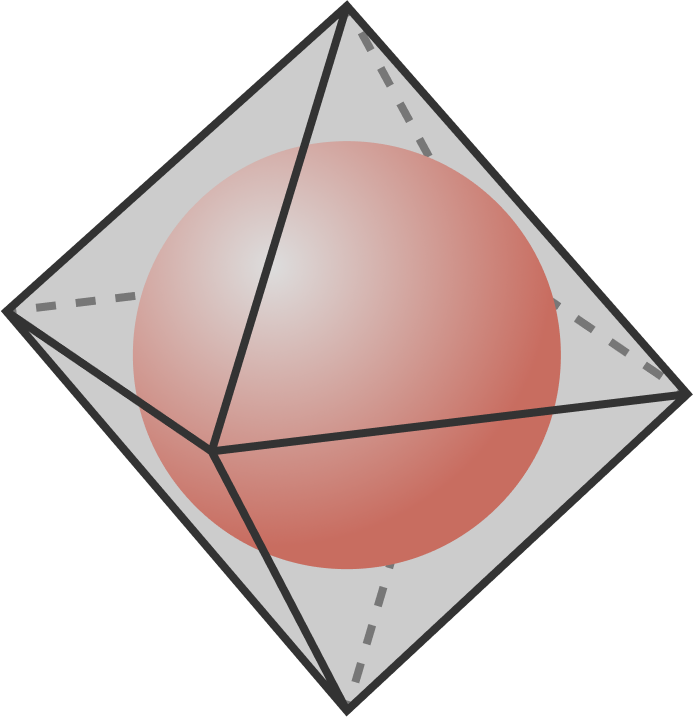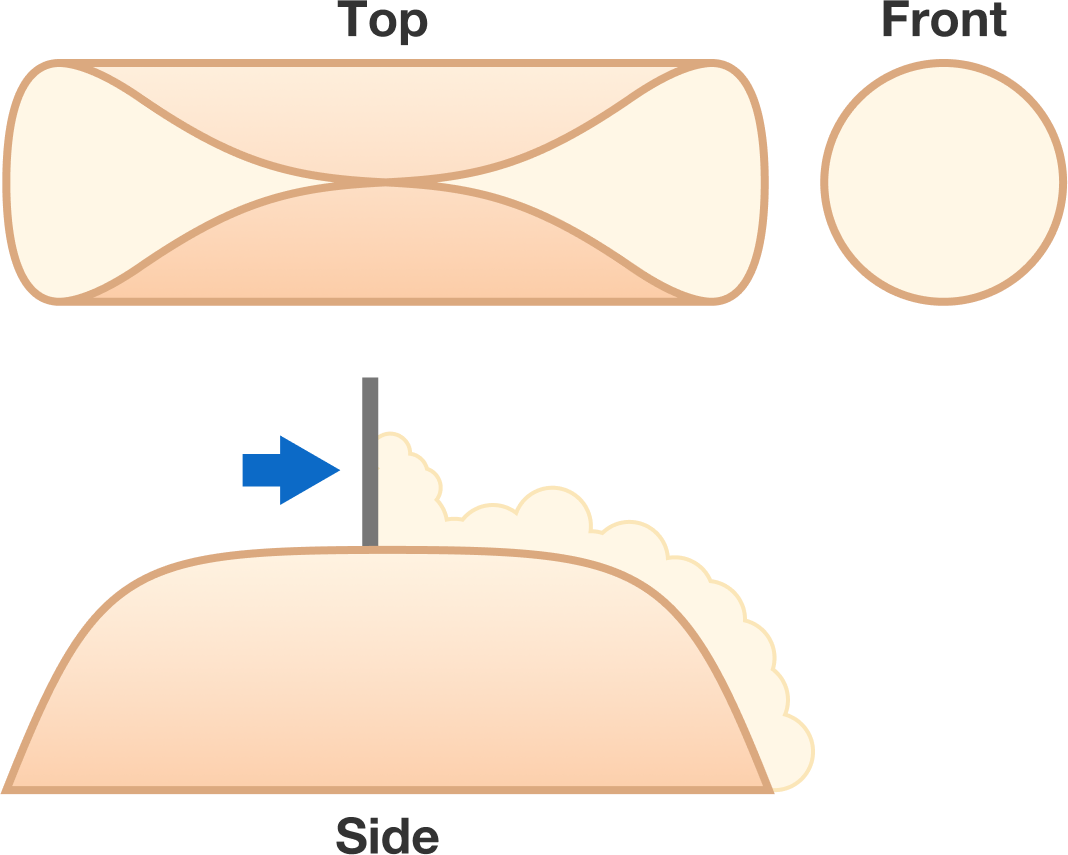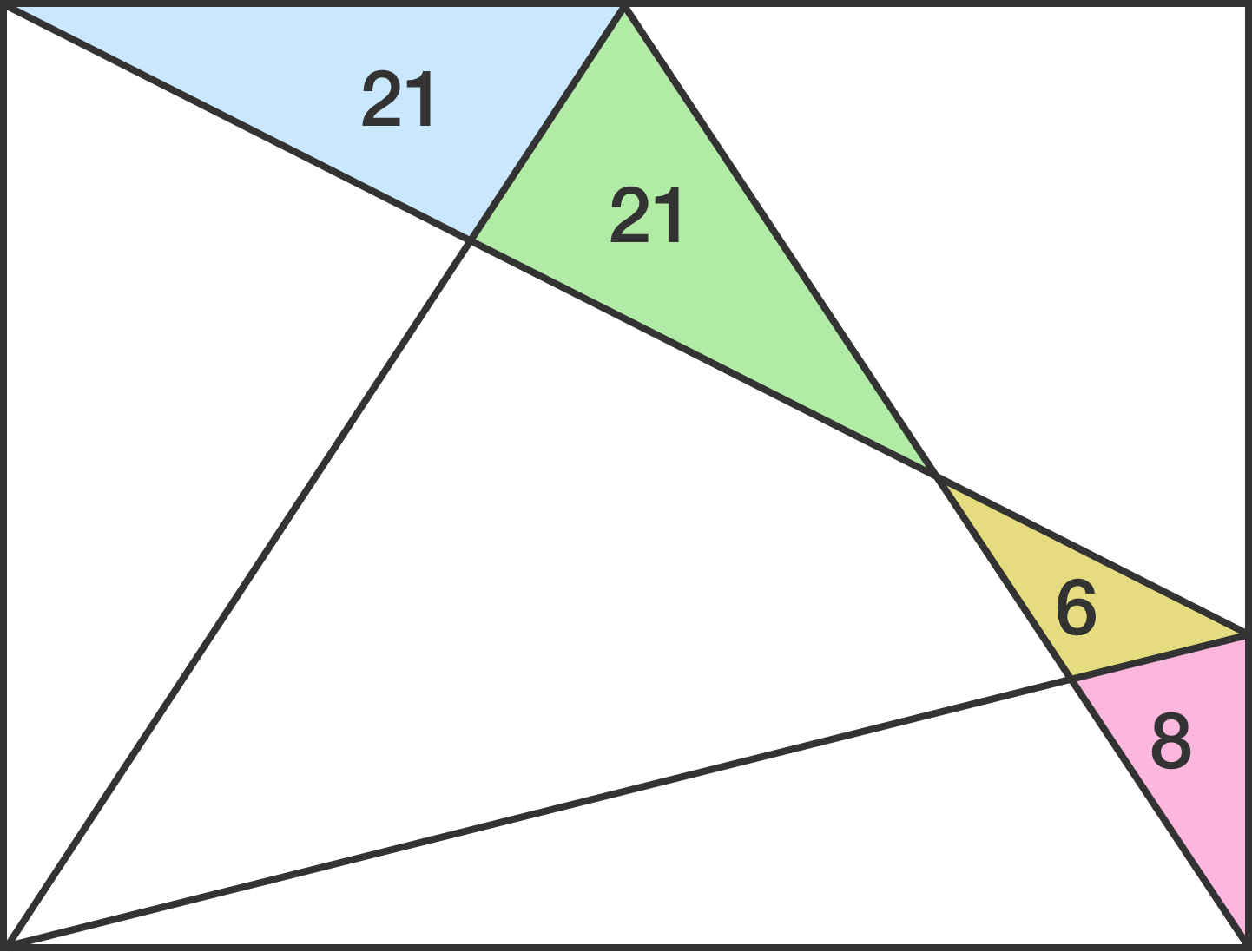# Problems of the Week

Contribute a problemA sphere is inscribed in a regular octahedron.

What is the ratio of the sphere’s radius to the octahedron’s edge length?

Find the sum of all primes of the form $p=n^{n} + 1$, where $p<10^{19}$ and $n$ is a positive integer.

Here is how to make the ideal mathematical cannoli:

1. Take a unit circle made of thin dough.
2. Wrap it around a cylinder of radius $\frac{1}{\pi}$ so the ends just touch.
3. Bake until crisp and then fill with delicious cream.
4. Scrape off the excess filling using a straight edge held perpendicular to the long axis and touching symmetric points of the edges as you scrape.

What is the volume of the filling in the cannoli?Note: Top, front, and side views of the cannoli are shown. Since the excess filling has been scraped away, no filling is visible in the side view. Bon appétit!

Given the areas of the triangles, find the area of this rectangle.From the AM-GM inequality, we know that the arithmetic mean (AM) of a list of non-negative real numbers is always greater than or equal to the geometric mean (GM). But the inequality doesn't tell us just how much larger the AM is.

Given a list of $n$ random real numbers chosen uniformly and independently in the range $[a_0,a_1],$ where $0\le a_0 find $\text{E}\left[\frac{\text{AM}}{\text{GM}}\right]$ in terms of $n,a_0,a_1.$

Then find $\displaystyle \lim\limits_{\overset{a_0 \to 0}{a_1 \to \infty}} \text{E}\left[\frac{\text{AM}}{\text{GM}}\right]$.

If the formula is of the form $\large\frac{n^n}{\left(An-B\right){\left(n-1\right)}^{n-C}},$ where $A,B,C$ are positive integers, give your answer as $A+B+C.$

Note: The notation $\text{E}[X]$ is the expected value of the random variable $X.$

×